Back to Assignments

A100 -- Dan Swearingen Homework 7
The Last Homework (if you don't count the final)
Due Tuesday, December 3 by the beginning of class

Page 311, Questions For Review:

6. Why must the interior of the Sun be so hot?

The Sun must be hot or it would collapse under its own gravity. The Sun's gravity compresses its core causing it to heat until its pressure balances the compression force. This balance is called hydrostatic equalibrium. If the Sun's core was not hot the Sun would shrink smaller and smaller under its own gravity and would eventually become and object you'll learn about in A105!

17. What is meant by solar neutrinos? Why do astronomers say there is a discrepancy? Why is the discrepancy of interest?

As hydrogen is converted into helium during thrmonuclear fusion, sub-atomic particles are created as by-products of this process. These particles are called neutrinos and neutrinos from the Sun are called solar neutrinos.

The number of neutrinos from the Sun detected on Earth is too small by a factor of two or three. Astronomers feel this is a serious problem because we can calculate how many neutrinos must be produced during nuclear fusion in the Sun.

This problem is of interest because it tells us that there must be a weakness in our understanding of the nature of neutrinos or of our understanding of the fusion process in the Sun.

Page 312, Thought Questions:

3. In this problem you will calculate approximately the temperature of the Sun's core. You can do it either step by step, or by writing out all the algebra to obtain a final result. You will need the following ideas:

(a) The pressure in a gas is given by the ideal gas law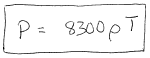.

(b) The density is a body's mass per volume, which for a sphere is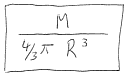.

(c) The pressure force from the interior is P x A, where A is the area over which the pressure acts. You can take that as the sun's cross-section,. Thus the pressure force is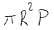.

Finally, you need to invoke hydrostatic equalibrium: "Pressure forces must balance gravitational forces." Approximate the gravitational force holding the Sun together by assuming it is split into two equal halves and apply Newton's law of gravity to calculate the force between the halves. Assume they are separated by one solar radius.

combining (a) and (b) into (c) we get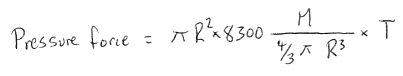To calculate the gravity force the we model the situation as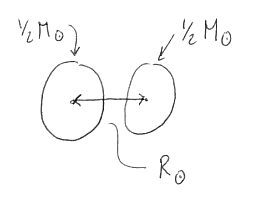Plugging into Newton's law of gravity we get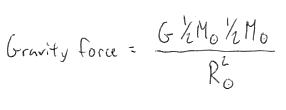Hydrostatic Equalibrium means that we must equate the Gravity force with the Pressure force so we can write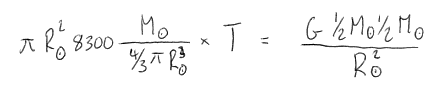Before we go further, lots of stuff cancels out: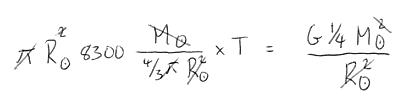Solving for T we have: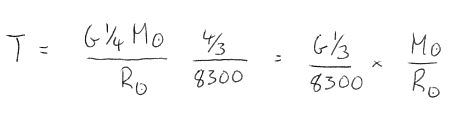Plugging in the appropriate numbers from the back of the book we haveConsidering the crudeness of the calculation we are in the ballpark.

Go to the website:

http://antwrp.gsfc.nasa.gov/apod/archivepix.html

this is an archive of NASA's Astronomy Picture of the Day website at

http://antwrp.gsfc.nasa.gov/apod/astropix.html

At the archive, find a page of the day which interests you and write a half page description (in your own words) of its topic and some of the resources linked to that page.

Places to access the web: Here on campus at the various computer labs, from home with your PC and AOL or connection to school, and the Monroe County Library has public web terminals.

Ice at the Lunar South Pole (December 4, 1996)

Explanation: Ice on the Moon? The prospecting Clementine spacecraft may well have discovered it. In 1994, Clementine spent 70 days in lunar orbit mapping the Moon's surface.

Like I said in class: I'm really excited about the discovery of quantities of ice on the moon. However, I wonder why this was a DoD mission?

Back to Assignments